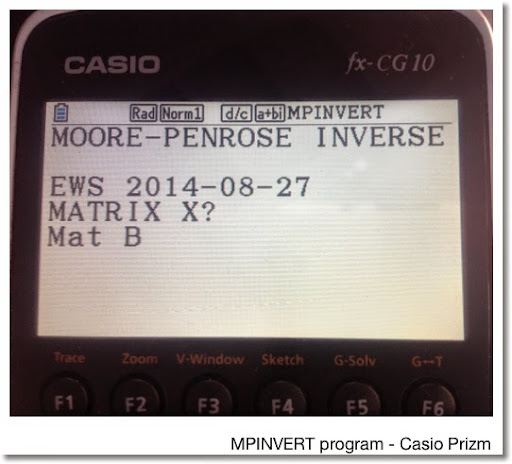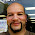## Friday, August 29, 2014

### Moore-Penrose Inverse of a MatrixMoore-Penrose Inverse of a Matrix
(E.H. Moore (1920), Arne Bjerhammar and Roger Penrose (1955))

Programs for the HP Prime and Casio Prizm (can be used for fx-9860g II and fx-9750g II) included

Also known as a pseudoinverse, the Moore-Penrose inverse of a matrix, denoted by A^+ (capital A with a supersubscript of a plus sign), is an inverse of matrix. Different from the "true" matrix inverse, the Moore-Penrose inverse allows for non-square matrices. Primarily, the Moore-Penrose inverses are calculated is assist in solving linear least-square equations.

Properties: Moore-Penrose Inverse

A A^+ A = A
A^+ A A^+ = A^+
(A A^+)^T = A
(A^+ A)^T = A^+

A^+ is unique

Calculation

One method of calculating the Moore-Penrose Inverse is the following:

For matrix A of size m × n:

Let r = row rank of A (number of linearly independent rows)
Let c = column rank of A (number of linearly independent columns)
Usually r = c
The matrix rank, denoted rank(A) = min(r, c)

If r ≤ c and rank(A) = r,
Then A^+ = A^T (A A^T)^-1

If r ≥ c and rank(A) = c,
Then A^+ = (A^T A)^-1 A^T

The programs listed on this blog entry assume the first conditions of each of the test are met, since usually, r = c.

Sources

Schott, James R. "Matrix Analysis for Statistics: 2nd Edition". Wiley & Sons. Hoboken, NJ. 2005

Wikipedia article, retrieved 8/29/2014 - URL:
http://en.m.wikipedia.org/wiki/Moore–Penrose_pseudoinverse

HP Prime Program MPINVERT

Input: MPINVERT(matrix). Execute this from Home or CAS screen.

Program:
EXPORT MPINVERT(mtx)
BEGIN
// 2014-08-27 EWS
// Moore-Penrose Matrix Inverse
LOCAL r,c,d,n;
d:=SIZE(mtx);
r:=d(1);
c:=d(2);
n:=RANK(mtx);
CASE
IF n==c THEN
RETURN (TRN(mtx)*mtx)^-1*mtx;
END;
IF n==r THEN
RETURN TRN(mtx)*(mtx*TRN(mtx))^-1;
END;
DEFAULT
RETURN "No Solution Found";
END;

END;

Casio Prizm Program MPINVERT

The program prompts for the matrix. Since the Prizm does not have a matrix rank command, it is determined by use of the rref command and pair of For loops. This program should work verbatim on the fx-9860g II and fx-9750g II.

The matrix is stored in Mat X. List 1 and Mat Y are used for calculation. The result is stored in Mat Z.

If you are using a TI-84, you would probably use an approach similar to what is presented:

Program MPINVERT
"MOORE-PENROSE INVERSE"
"EWS 2014-08-27"
"MATRIX X"? → Mat X
Dim Mat X→List 1
List 1 → R
List 1 → C
Rref Mat X → Mat Y
0 → T
For 1 → I To R
For 1 → J To C
If Mat Y[I, J] ≠ 0
Then
T + 1 → T
Break
IfEnd
Next
Next
If T = R
Then
Trn Mat X * (Mat X * Trn Mat X) ^ -1 → Mat Z
"Mat Z"
Mat Z ◢ // display
Stop
IfEnd
If T = C
Then
(Trn Mat X * Mat X) ^ -1 * Trn Mat X → Mat Z
"Mat Z"
Mat Z ◢ // display
Stop
IfEnd
"NO INVERSE"

Examples

Matrix:
[ [ 1, 2, 3 ] [ 3, 4, 0 ] ]

Moore-Penrose Inverse:
[ [ -8/229, 31/229 ] [ 6/229, 34/229 ] [ 75/229, -33/229 ] ]

Matrix:
[ [7, 4, 6, -7] [-1, 5, 3, 3] ]

Moore-Penrose Inverse: (to four decimal places)
[ [0.0489, -0.0338] [0.0194, 0.1092] [0.0360, 0.0600] [-0.0520, 0.0800] ]

This blog is property of Edward Shore. 2014

1.I enjoyed your blog.I used to CASIO calculator.Ever function in this wiseness manage..
Call for Inquiry at any time or Free Demo..
Mobile: +91-9799950666 /555/444
loan calculator software | Commercial Loan Software

2.Thanks cyrus!

3."Strength and soundness of business depends on the availability of finance and competency with which it is used. The abundance of finance can do wonders and its scarcity can ruin even a well established business."!!

Micro Finance Software

### Retro Review: Radio Shack EC-2024

Retro Review:  Radio Shack EC-2024 Quick Facts Company:  Radio Shack Years in Production: Around 1994 Original Price: \$24.99 Bat...